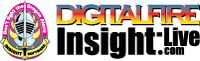•The secret to cool bodies and glazes is a lot of testing.
•The secret to know what to test is material and chemistry knowledge.
•The secret to learning from testing is documentation.
•The place to test, do the chemistry and document is an account at https://insight-live.com
•The place to get the knowledge is https://digitalfire.com

Sign-up at https://insight-live.com today.

# Ball Clay FX (CA1)

 Oxide Analysis Formula CaO 0.06% 0.004 MgO 0.77% 0.078 K2O 2.88% 0.124 Na2O 0.16% 0.010 TiO2 1.27% 0.065 Al2O3 25.10% 1.000 SiO2 61.50% 4.158 Fe2O3 1.00% 0.025 LOI 7.29 Oxide Weight 376.87 Formula Weight 406.51

## XML for Import into INSIGHT

<?xml version="1.0" encoding="UTF-8"?> <material name="Ball Clay FX (CA1)" descrip="" searchkey="" loi="0.00" casnumber=""> <oxides> <oxide symbol="CaO" name="Calcium Oxide, Calcia" status="" percent="0.060" tolerance=""/> <oxide symbol="MgO" name="Magnesium Oxide, Magnesia" status="" percent="0.770" tolerance=""/> <oxide symbol="K2O" name="Potassium Oxide" status="" percent="2.880" tolerance=""/> <oxide symbol="Na2O" name="Sodium Oxide, Soda" status="" percent="0.160" tolerance=""/> <oxide symbol="TiO2" name="Titanium Dioxide, Titania" status="" percent="1.270" tolerance=""/> <oxide symbol="Al2O3" name="Aluminum Oxide, Alumina" status="" percent="25.100" tolerance=""/> <oxide symbol="SiO2" name="Silicon Dioxide, Silica" status="" percent="61.500" tolerance=""/> <oxide symbol="Fe2O3" name="Iron Oxide, Ferric Oxide" status="" percent="1.000" tolerance=""/> </oxides> <volatiles> <volatile symbol="LOI" name="Loss on Ignition" percent="7.290" tolerance=""/> </volatiles> </material>